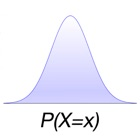Probability Distributions Calculator

• 검색 유형

모든 iPhone 애플리케이션 카테고리

모든 iPhone 게임 카테고리# Probability Distributions Calculator

0 0

## 스크린샷

설명

Easily calculate probabilities using the most common distribution models. The app gives you the value of a probability, the mean and variance.

Discrete models: Bernoulli, Binomial, Geometric, Pascal, Hipergeometric, Poisson.

Continuous models: Uniform, Exponential, Normal.